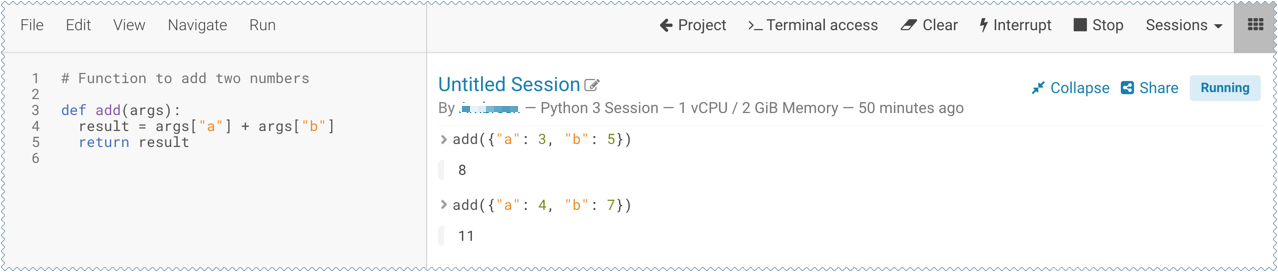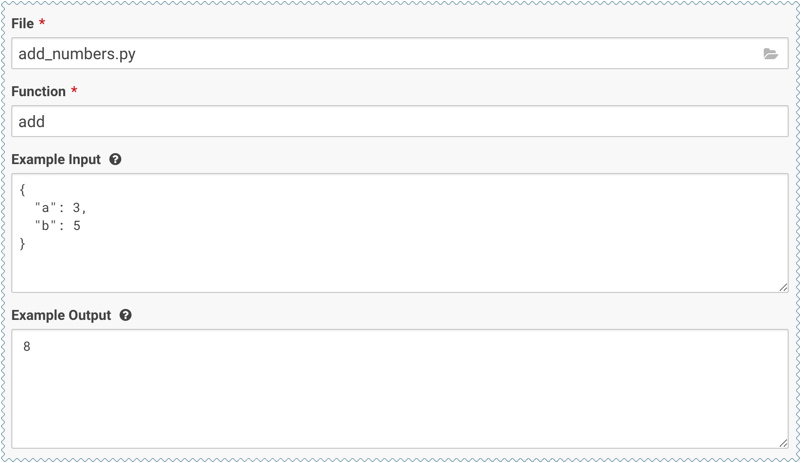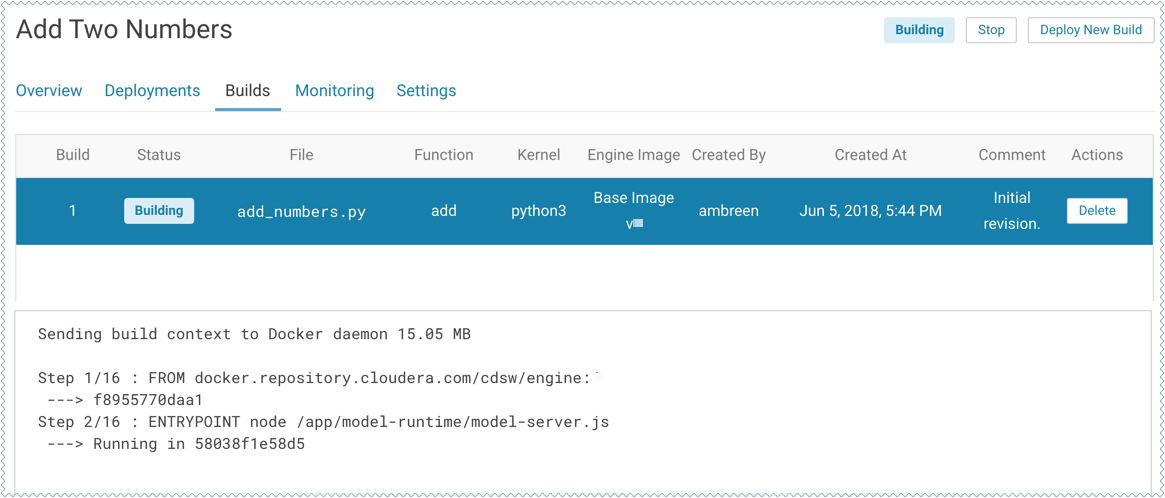# Creating and Deploying a Model

This topic describes a simple example of how to create and deploy a model using Cloudera Machine Learning.

Using Cloudera Machine Learning, you can create any function within a script and deploy it to a REST API. In a machine learning project, this will typically be a predict function that will accept an input and return a prediction based on the model's parameters.

For the purpose of this quick start demo we are going to create a very simple function that adds two numbers and deploy it as a model that returns the sum of the numbers. This function will accept two numbers in JSON format as input and return the sum.

1. Create a new project. Note that models are always created within the context of a project.
2. Click New Session and launch a new Python 3 session.
3. Create a new file within the project called `add_numbers.py`. This is the file where we define the function that will be called when the model is run. For example:

``````def add(args):
result = args["a"] + args["b"]
return result``````
4. Before deploying the model, test it by running the `add_numbers.py` script, and then calling the `add` function directly from the interactive workbench session. For example:
``add({"a": 3, "b": 5})``5. Deploy the `add` function to a REST endpoint.
1. Go to the project Overview page.
2. Click Models > New Model.
3. Give the model a Name and Description.
4. Enter details about the model that you want to build. In this case:
• Example Input: {"a": 3, "b": 5}
• Example Output: 85. Select the resources needed to run this model, including any replicas for load balancing.
6. Click Deploy Model.
6. Click on the model to go to its Overview page. Click Builds to track realtime progress as the model is built and deployed. This process essentially creates a Docker container where the model will live and serve requests.7. Once the model has been deployed, go back to the model Overview page and use the Test Model widget to make sure the model works as expected.

If you entered example input when creating the model, the Input field will be pre-populated with those values. Click Test. The result returned includes the output response from the model, as well as the ID of the replica that served the request.

Model response times depend largely on your model code. That is, how long it takes the model function to perform the computation needed to return a prediction. It is worth noting that model replicas can only process one request at a time. Concurrent requests will be queued until the model can process them.

To create and deploy a model using the API, follow this example:

This example demonstrates the use of the Models API. To run this example, first do the following:
1. Create a project with the Python template and a legacy engine.
2. Start a session.
3. Run `!pip3 install sklearn`
4. Run fit.py

The example script first obtains the project ID, then creates and deploys a model.

``````projects = client.list_projects(search_filter=json.dumps({"name": “<your project name>”}))
project = projects.projects # assuming only one project is returned by the above query
model_body = cmlapi.CreateModelRequest(project_id=project.id, name="Demo Model", description="A simple model")
model = client.create_model(model_body, project.id)
model_build_body = cmlapi.CreateModelBuildRequest(project_id=project.id, model_id=model.id, file_path="predict.py", function_name="predict", kernel="python3")
model_build = client.create_model_build(model_build_body, project.id, model.id)
while model_build.status not in [“built”, “build failed”]:
print(“waiting for model to build...”)
time.sleep(10)
model_build = client.get_model_build(project.id, model.id, model_build.id)
if model_build.status == “build failed”: